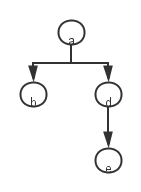•闪存
•博客
• 发言小组
• 投递新闻
• 提问博问
• 添加收藏
•文库

# java中HashTable的put这样使用是什么意思？

0悬赏园豆：30 [待解决问题]```public class Edge {
//Name of origin town
public Node origin;
//Name of destination town
public Node destination;
//Route weight to destination
public int weight;
//next possible route
public Edge next;
//constructor
public Edge(Node origin, Node destination, int weight) {
this.origin         = origin;
this.destination    = destination;
this.weight         = weight;
this.next         = null;
}

public Edge next(Edge edge) {
this.next = edge;
return this;
}
}```
`graph.routeTable.put(a, new Edge(a, b, 5).next(new Edge(a, d, 5).next(new Edge(a, e, 7))));`

new Edge(a,b,5).next().next()连着用.netx()是什么意思？ 请大家指教！

0

new Edge(a,b,5).next().next()

```1     public Edge next(Edge edge) {
2         this.next = edge;
3         return this;
4     }```

ThreeTree | 园豆：1490 (小虾三级) | 2014-04-18 18:46

graph.routeTable.put(a, new Edge(a, b, 5).next(new Edge(a, d, 5).next(new Edge(a, e, 7))));

0

new Edge(a, e, 7)这是创造了一个从a->e权重为7的边（我们叫这条边X1），

new Edge(a, d, 5).next(X1)这是创造了一个a->d权重为5的边（X2，并且X2可以通过this.next引用到X1），

new Edge(a, b, 5).next(X2)这是创造了一个a->b权重为5的边（X3，并且X3可以通过this.next引用到X2），

0

package com.test;

import java.util.HashMap;
import java.util.Map;
import java.util.Set;

public class TestHashMap {

public static void main(String[] args) {
Map map=new HashMap();
map.put("key1", "value1");
map.put("key2", "value2");
map.put("key3", "value3");
map.put("key4", "value4");
map.put("key5", "value5");
Set set=map.keySet();
for(Object key:set){
System.out.println(key+"="+map.get(key));
}

}

}

flab | 园豆：208 (菜鸟二级) | 2014-05-06 12:41
0graph.routeTable.put(a, new Edge(a, b, 5).next(new Edge(a, d, 5).next(new Edge(a, e, 7))));

hashtable.put(key,value);

key为 a

value为new Edge(a, b, 5).next(new Edge(a, d, 5).next(new Edge(a, e, 7)))

a到b重5 ，a到d也重5 ，a分出来两支，a到e重7，a到e中包含了a到d，所以d到e重2。

Even丶 | 园豆：218 (菜鸟二级) | 2014-05-12 11:12

您需要登录以后才能回答，未注册用户请先注册